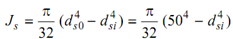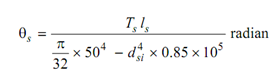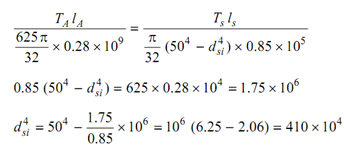## Find the inner diameter of the tubular steel shaft, Mechanical Engineering

Assignment Help:

Find the inner diameter of the tubular steel shaft:

A solid aluminium shaft having 1 m long & of 50 mm diameter is to be replaced by a tubular steel shaft of the similar length and similar outside diameter so that either shaft might carry the same torque and contain the similar angle of twist, over the overall length. What should be the inner diameter of the tubular steel shaft?

Modulus of rigidity of steel might be taken as 0.85 × 105 N/mm2 and that of aluminium as 0.28 × 105 N/mm2.

Solution

Solid Aluminium Shaft lA = 1000 mm

dA = 50 mm

GA = 0.28 × 105 N/mm2

Tabular Steel Shaft

Ls = 100 cm

Ds0 = 50 mm,  dsi = ?

Gs = 0.85 × 105 N/mm2

Here,   TA = Ts and θA = θs

J A=  π (d A)4  = (π/32) × 504  = 6.25 π × 106 / 32 mm4We know,

T/J  = G θ/l or θ = T l/JG

Therefore,

θA  = (TA lA   /  ((625 π/32) × 0.28 × 10) ) radianSince θA = θs and also TA  = Ts , lA  = lsdsi  = 45. 243 mm

#### Steam generator piping, Q. Steam Generator Piping? Control valves shall...

Q. Steam Generator Piping? Control valves shall be Fisher or Purchaser-approved equal. All valves shall be detailed by size, rating, make, model number, and materials Two

#### Answer, give me an example of law of superposition

give me an example of law of superposition

#### Stress distribution diagram, why stress distribution diagram is different f...

why stress distribution diagram is different for I section, T section andcircular section?

#### Thermodynamics, microscopic and macroscopic view points

microscopic and macroscopic view points

#### law of gearing, explain the law of gearin

explain the law of gearing

#### Find out the moment of inertia of a semicircle, Find out the moment of ine...

Find out the moment of inertia  of a semicircle: Find out the M. I. of a semicircle ABM around its periphery AB and also around centroidal axes x and y as illustrated in Figur

#### Thermodynamics assignment, The steam that leaves the super-heater is expand...

The steam that leaves the super-heater is expanded from 130bar at 535°C to 25.2bar at 310°C in a high pressure turbine. The steam from the exit of the HP turbine is reheated to 535

Q. Ultrasound Bolt Load Monitoring? Ultrasound measurement of bolt stresses, using ‘Bolt Scope', should be used on: • All bolts tightened by tensioning. • All bolts in hy

#### Find minimum thickness, A 1.2 m diameter steel pipe carries oil of relative...

A 1.2 m diameter steel pipe carries oil of relative density 0.822 under a head of 70 m of oil. What minimum thickness of 120 MPa (yield stress) steel would be required for a safety

#### Evaluate the velocity ratio, (a) A geometrically similar model of spillway ...

(a) A geometrically similar model of spillway is to be laid to a scale of 1 in 50. Evaluate the velocity ratio, discharge ratio and acceleration ratio. (b) If model prototype ra